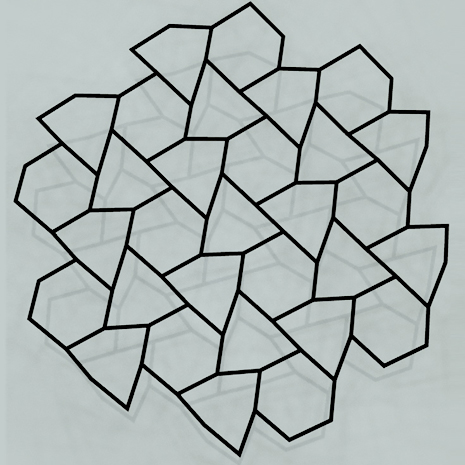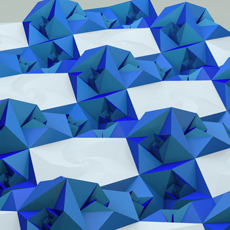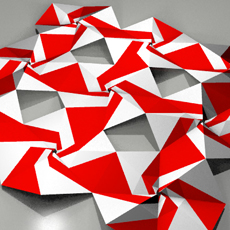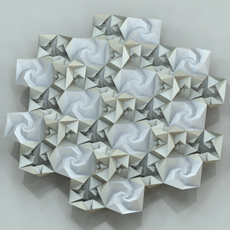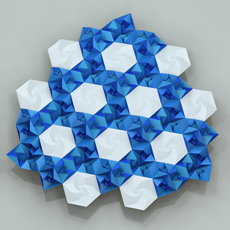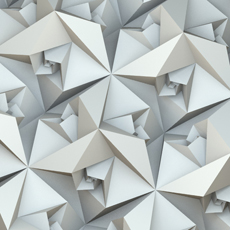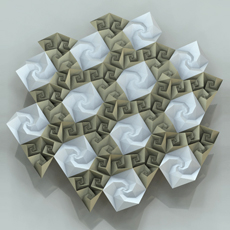Spidron System
 1. General Concept 2. Tilings 3. Reliefs 4. Fractals and Variations 5. Polyhedra 6. Dissections 7. Spacefilling 8. Transformations 9. Extra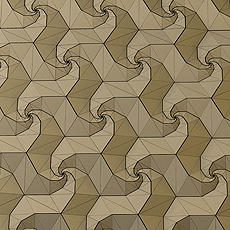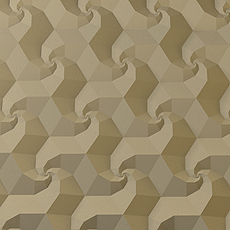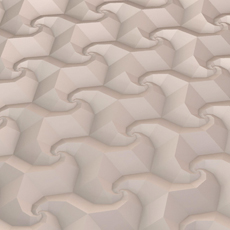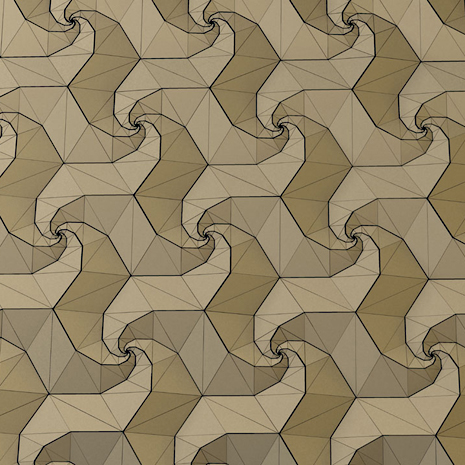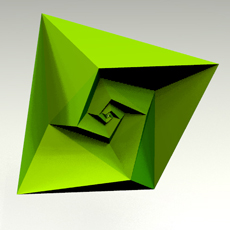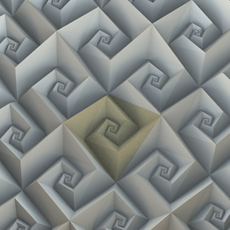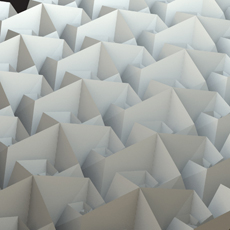If several spidron nests are connected to each other, we get a special accordion shape - I called spidron relief - that deforms in vertices around centre points arranged in a grid, which can be described in all its transitional states as well as in motion using a mathematical algorithm. The system of equations indicates that with respect to the edges, the movement is, technically speaking, simultaneously recursive, which means that on the one hand, the angle between the edges of a specific spidron ring and the base plane can only be calculated from the angle between a corresponding edge on a neighbouring spidron ring and the base plane, and on the other hand, the rotation of the same edges with respect to the centre of the spidron nest can also be calculated in the same way.